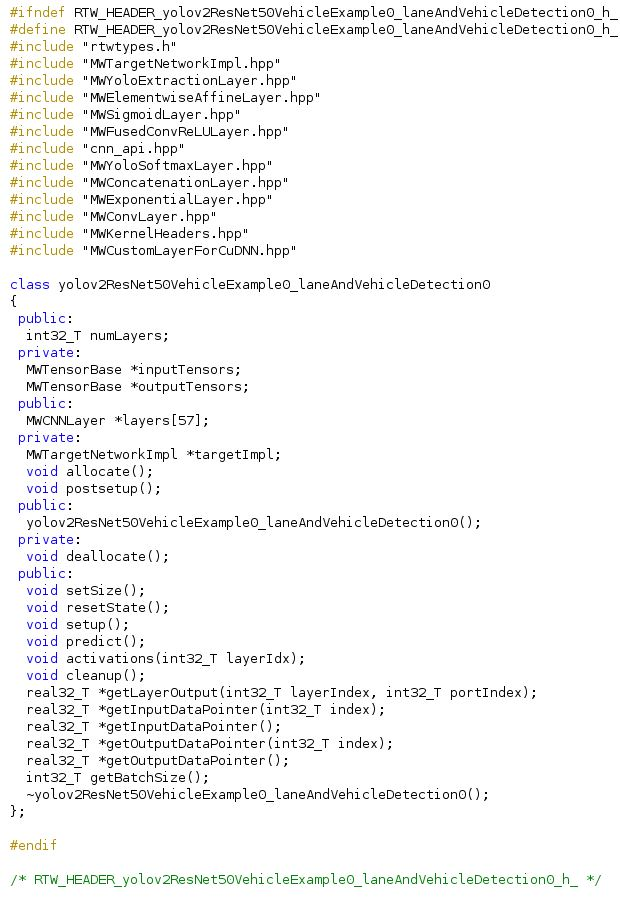# Code Generation for a Deep Learning Simulink Model that Performs Lane and Vehicle Detection

This example shows how to develop a CUDA® application from a Simulink® model that performs lane and vehicle detection using convolutional neural networks (CNN). This example takes the frames of a traffic video as an input, outputs two lane boundaries that correspond to the left and right lanes of the ego vehicle, and detects vehicles in the frame. This example uses the pretrained lane detection network from the Lane Detection Optimized with GPU Coder example of the GPU Coder Toolbox™. For more information, see Lane Detection Optimized with GPU Coder. This example also uses the pretrained vehicle detection network from the Object Detection Using YOLO v2 Deep Learning example of the Computer Vision toolbox™. For more information, see Object Detection Using YOLO v2 Deep Learning (Computer Vision Toolbox).

This example illustrates the following concepts:

• Model the lane detection application in Simulink. First the traffic video is preprocessed by resizing to 227x227x3 and multiplication by a constant factor of 255. Subsequently, it is processed by the pretrained network loaded in the `Predict` block from the Deep Learning Toolbox™. Finally, if the left and right lane boundaries are detected, the parabolic coefficients to model the trajectories of the lane boundaries are obtained.

• Model the vehicle detection application in Simulink. The traffic video is processed by a pretrained YOLO v2 detector. This network detects vehicles in the video and outputs the coordinates of the bounding boxes for these vehicles and their confidence score.

• Configure the model for code generation.

• Generate a CUDA executable for the Simulink model.

### Verify GPU Environment

To verify that the compilers and libraries necessary for running this example are set up correctly, use the `coder.checkGpuInstall` function.

```envCfg = coder.gpuEnvConfig('host'); envCfg.DeepLibTarget = 'cudnn'; envCfg.DeepCodegen = 1; envCfg.Quiet = 1; coder.checkGpuInstall(envCfg); ```

### Algorithmic Workflow

The block diagram for the algorithmic workflow of the Simulink model is shown.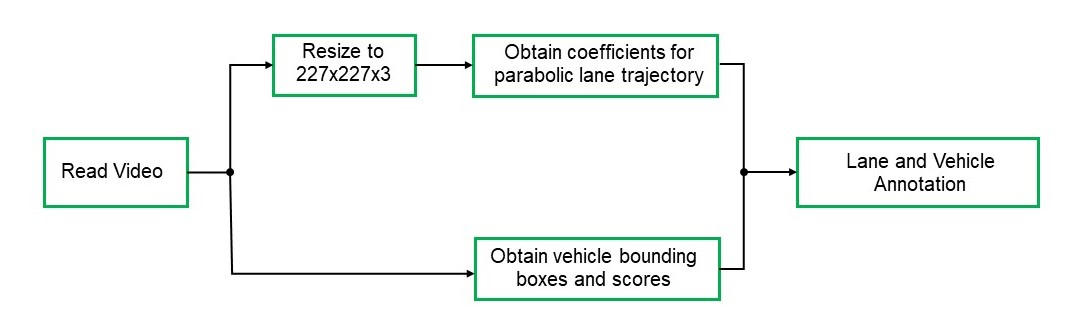### Get Pretrained Lane and Vehicle Detection Networks

This example uses the `trainedLaneNet` and `yolov2ResNet50VehicleExample` MAT-files containing the pretrained networks. The files are approximately 143MB and 98MB in size, respectively. Download the files from the MathWorks website.

```lanenetFile = matlab.internal.examples.downloadSupportFile('gpucoder/cnn_models/lane_detection','trainedLaneNet.mat'); vehiclenetFile = matlab.internal.examples.downloadSupportFile('vision/data','yolov2ResNet50VehicleExample.mat'); ```

To test the model, the example uses the Caltech lanes dataset. The file is approximately 16 MB in size. Download the files from the MathWorks website.

```mediaFile = matlab.internal.examples.downloadSupportFile('gpucoder/media','caltech_washington1.avi'); ```

### Lane and Vehicle Detection Simulink Model

The Simulink model for performing lane and vehicle detection on the traffic video is shown. When the model runs, the `Video Viewer` block displays the traffic video with lane and vehicle annotations.

```open_system('laneAndVehicleDetection'); ```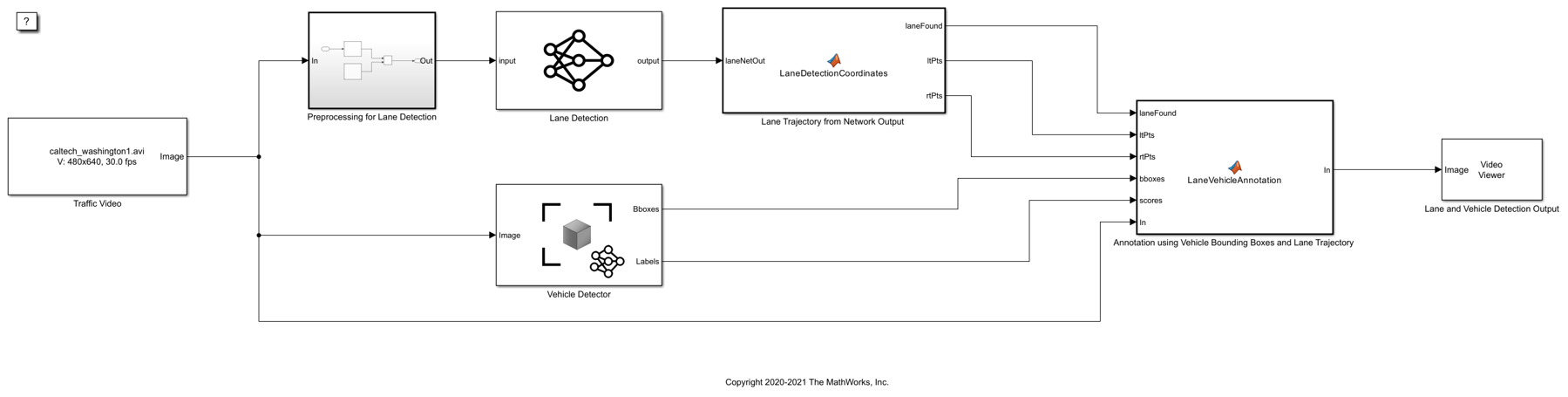Set the file paths of the dowloaded network model in the predict and detector blocks of the Simulink model. Set the location of the test video to be loaded by the Simulink model.

```set_param('laneAndVehicleDetection/Lane Detection','NetworkFilePath',lanenetFile) set_param('laneAndVehicleDetection/Vehicle Detector','DetectorFilePath',vehiclenetFile) set_param('laneAndVehicleDetection/Traffic Video','inputFileName',mediaFile) ```

### Lane Detection

The `Predict` block loads the pretrained lane detection network from the `trainedLaneNet.mat` file. This network takes an image as an input and outputs two lane boundaries that correspond to the left and right lanes of the ego vehicle. Each lane boundary is represented by the parabolic equation:

`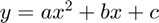`

Here y is the lateral offset and x is the longitudinal distance from the vehicle. The network outputs the three parameters a, b, and c per lane. The network architecture is similar to `AlexNet` except that the last few layers are replaced by a smaller fully connected layer and regression output layer. The `LaneDetectionCoordinates` MATLAB function block defines a function `lane_detection_coordinates` that takes the output from the predict block and outputs three parameters i.e. `laneFound`, `ltPts` and `rtPts`. Thresholding is used to determine if both left and right lane boundaries are both found. If both are found, `laneFound` is set to be true and the trajectories of the boundaries are calculated and stored in `ltPts` and `rtPts` respectively.

```type lane_detection_coordinates ```
```function [laneFound,ltPts,rtPts] = lane_detection_coordinates(laneNetOut) % Copyright 2020-2021 The MathWorks, Inc. persistent laneCoeffMeans; if isempty(laneCoeffMeans) laneCoeffMeans = [-0.0002,0.0002,1.4740,-0.0002,0.0045,-1.3787]; end persistent laneCoeffStds; if isempty(laneCoeffStds) laneCoeffStds = [0.0030,0.0766,0.6313,0.0026,0.0736,0.9846]; end params = laneNetOut .* laneCoeffStds + laneCoeffMeans; % 'c' should be more than 0.5 for it to be a right lane isRightLaneFound = abs(params(6)) > 0.5; isLeftLaneFound = abs(params(3)) > 0.5; persistent vehicleXPoints; if isempty(vehicleXPoints) vehicleXPoints = 3:30; %meters, ahead of the sensor end ltPts = coder.nullcopy(zeros(28,2,'single')); rtPts = coder.nullcopy(zeros(28,2,'single')); if isRightLaneFound && isLeftLaneFound rtBoundary = params(4:6); rt_y = computeBoundaryModel(rtBoundary, vehicleXPoints); ltBoundary = params(1:3); lt_y = computeBoundaryModel(ltBoundary, vehicleXPoints); % Visualize lane boundaries of the ego vehicle tform = get_tformToImage; % Map vehicle to image coordinates ltPts = tform.transformPointsInverse([vehicleXPoints', lt_y']); rtPts = tform.transformPointsInverse([vehicleXPoints', rt_y']); laneFound = true; else laneFound = false; end end ```

### Vehicle Detection

A YOLO v2 object detection network is composed of two subnetworks: a feature extraction network followed by a detection network. This pretrained network uses a `ResNet-50` for feature extraction. The detection sub-network is a small CNN compared to the feature extraction network and is composed of a few convolutional layers and layers specific to YOLO v2. The Simulink model performs vehicle detection using the `Object Detector` block from the Computer Vision Toolbox( TM). This block takes an image as input and outputs the bounding box coordinates along with the confidence scores for vehicles in the image.

### Annotation of Vehicle Bounding Boxes and Lane Trajectory in Traffic Video

The `LaneVehicleAnnotation` MATLAB function block defines a function `lane_vehicle_annotation` which annotates the vehicle bounding boxes along with the confidence scores. Also, if `laneFound` is true, then the left and right lane boundaries stored in `ltPts` and `rtPts` are annotated in the traffic video.

```type lane_vehicle_annotation ```
```function In = lane_vehicle_annotation(laneFound,ltPts,rtPts,bboxes,scores,In) % Copyright 2020-2021 The MathWorks, Inc. if ~isempty(bboxes) In = insertObjectAnnotation(In, 'rectangle',bboxes,scores); end pts = coder.nullcopy(zeros(28, 4, 'single')); if laneFound prevpt = [ltPts(1,1) ltPts(1,2)]; for k = 2:1:28 pts(k,1:4) = [prevpt ltPts(k,1) ltPts(k,2)]; prevpt = [ltPts(k,1) ltPts(k,2)]; end In = insertShape(In, 'Line', pts, 'LineWidth', 2); prevpt = [rtPts(1,1) rtPts(1,2)]; for k = 2:1:28 pts(k,1:4) = [prevpt rtPts(k,1) rtPts(k,2)]; prevpt = [rtPts(k,1) rtPts(k,2)]; end In = insertShape(In, 'Line', pts, 'LineWidth', 2); In = insertMarker(In, ltPts); In = insertMarker(In, rtPts); end end ```

### Run the Simulation

Open Configuration Parameters dialog box.

In Simulation Target pane, select GPU acceleration. In the Deep Learning group, select the target library as cuDNN.

```set_param(bdroot,'GPUAcceleration','on'); set_param(bdroot,'SimDLTargetLibrary','cudnn'); set_param(bdroot,'DLTargetLibrary','cudnn'); ```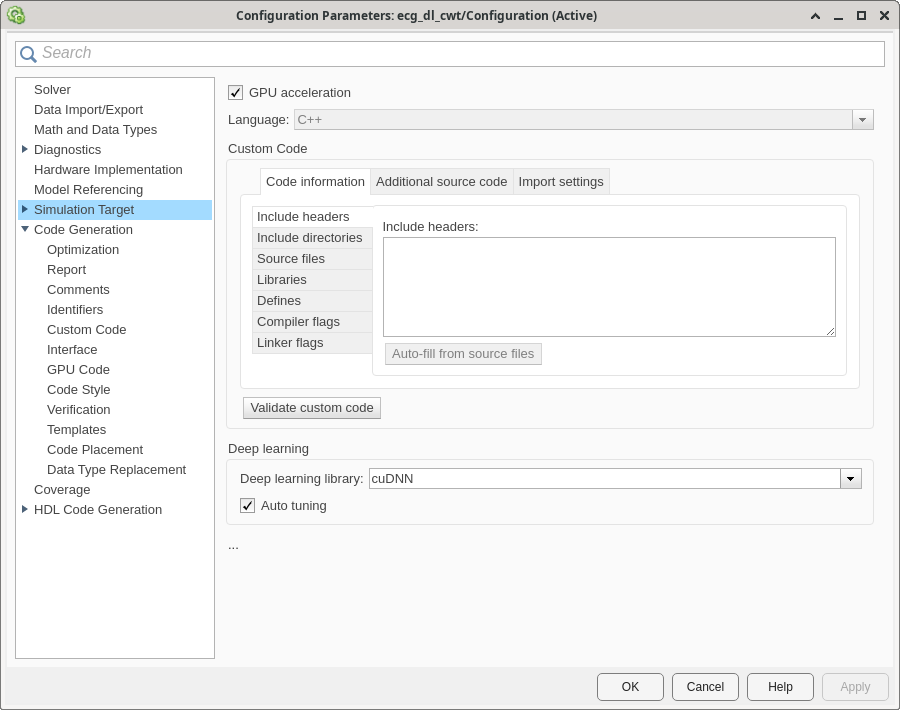To verify the lane and vehicle detection algorithms and display the lane trajectories, vehicle bounding boxes and scores for the traffic video loaded in the Simulink model, run the simulation.

```set_param('laneAndVehicleDetection', 'SimulationMode', 'Normal'); sim('laneAndVehicleDetection'); ```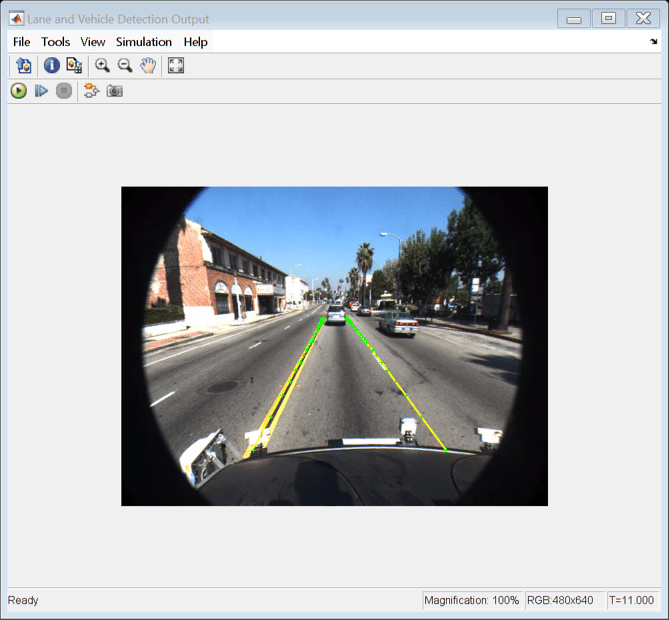### Generate and Build the Simulink Model

In Code Generation pane, select the Language as C++ and enable Generate GPU code.

```set_param(bdroot,'TargetLang','C++'); set_param(bdroot,'GenerateGPUCode','CUDA'); set_param(bdroot,'GenCodeOnly','on'); if ispc set_param(bdroot,'ProdHWDeviceType','Intel->x86-64 (Windows64)'); else set_param(bdroot,'ProdHWDeviceType','Intel->x86-64 (Linux 64)'); end set_param(bdroot,'ProdLongLongMode','on'); ```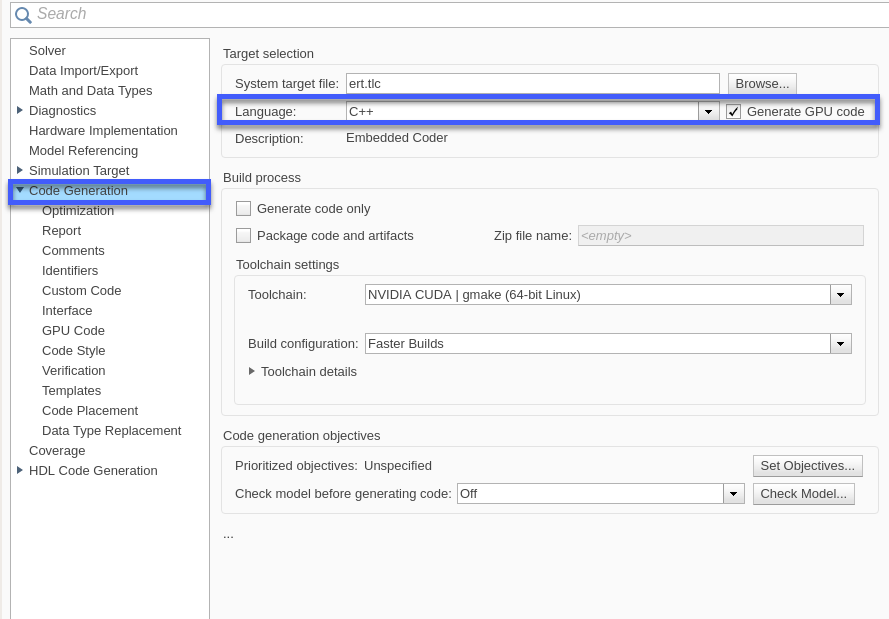In the subcategory Libraries of the Code Generation > GPU Code pane, enable cuBLAS, cuSOLVER and cuFFT.

```set_param(bdroot,'GPUcuBLAS','on'); set_param(bdroot,'GPUcuSOLVER','on'); set_param(bdroot,'GPUcuFFT','on'); ```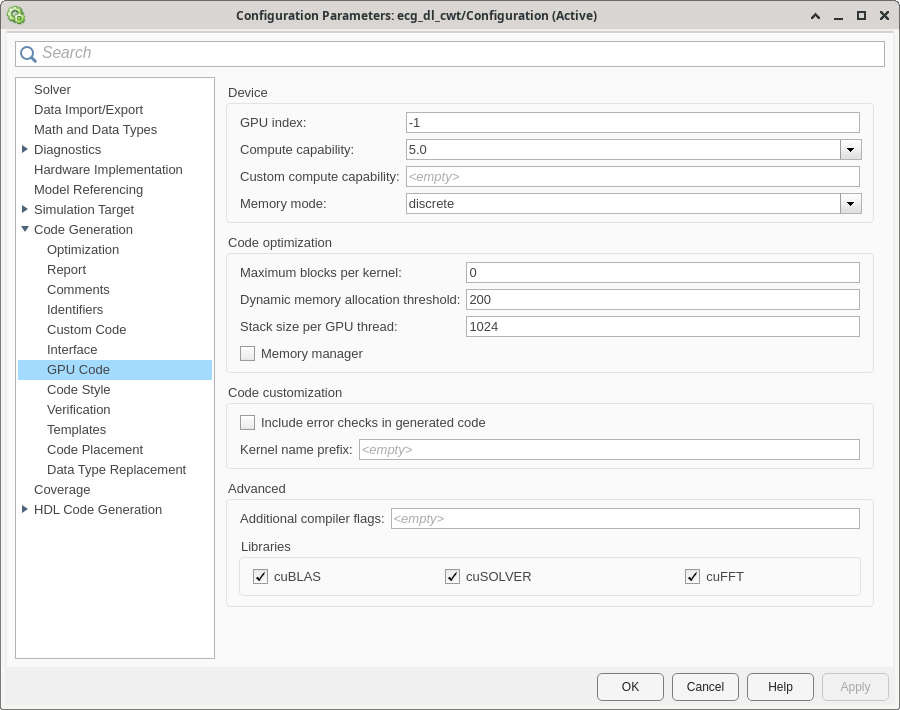Generate and build the Simulink model on the host GPU by using the `slbuild` command. The code generator places the files in a build folder, a subfolder named `laneAndVehicleDetection_ert_rtw` under your current working folder.

```status = evalc("slbuild('laneAndVehicleDetection')"); ```

### Generated CUDA Code

The subfolder named `laneAndVehicleDetection_ert_rtw` contains the generated C++ codes corresponding to the different blocks in the Simulink model and the specific operations being performed in those blocks. For example, the file `trainedLaneNet0_laneAndVehicleDetection0.h` contains the C++ class which contains attributes and member functions representing the pretrained lane detection network.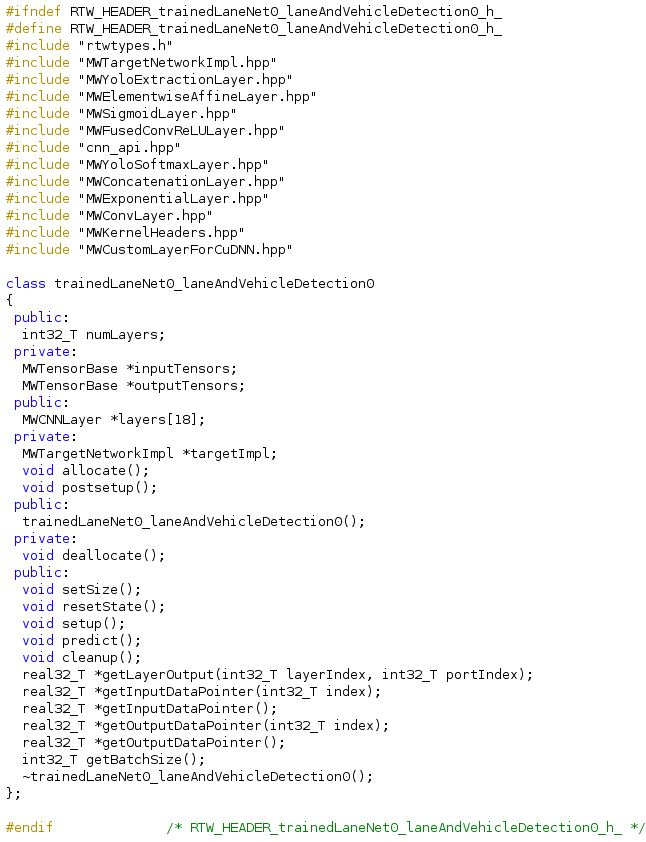Similarly, the file `yolov2ResNet50VehicleExample0_laneAndVehicleDetection0.h` contains the C++ class representing the pretrained YOLO v2 detection network.